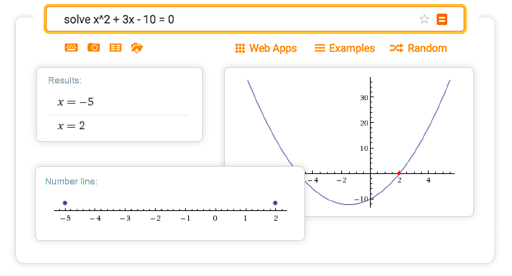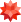# Online Equation Solver

## More than just an online equation solver

Wolfram|Alpha is a great tool for finding polynomial roots and solving systems of equations. It also factors polynomials, plots polynomial solution sets and inequalities and more.## Tips for entering queries

Enter your queries using plain English. To avoid ambiguous queries, make sure to use parentheses where necessary. Here are some examples illustrating how to formulate queries.

## About solving equations

A value c is said to be a root of a polynomial p(x) if p(c)=0. The largest exponent of x appearing in p(x) is called the degree of p. If p(x) has degree n, then it is well known that there are n roots, once one takes into account multiplicity. To understand what is meant by multiplicity, take, for example, x^2-6x+9=(x-3)^2=(x-3)(x-3). This polynomial is considered to have two roots, both equal to 3.

One learns about the 'factor theorem,' typically in a second course on algebra, as a way to find all roots that are rational numbers. One also learns how to find roots of all quadratic polynomials, using square roots (arising from the discriminant) when necessary. There are more advanced formulas for expressing roots of cubic and quartic polynomials, and also a number of numeric methods for approximating roots of arbitrary polynomials. These use methods from complex analysis as well as sophisticated numerical algorithms, and indeed, this is an area of ongoing research and development.

Systems of linear equations are often solved using Gaussian elimination or related methods. This too is typically encountered in secondary or college math curricula. More advanced methods are needed to find roots of simultaneous systems of nonlinear equations. Similar remarks hold for working with systems of inequalities: the linear case can be handled using methods covered in linear algebra courses, whereas higher-degree polynomial systems typically require more sophisticated computational tools.

## How Wolfram|Alpha solves equations

For equation solving, Wolfram|Alpha calls the Wolfram Language's Solve and Reduce functions, which contain a broad range of methods for all kinds of algebra, from basic linear and quadratic equations to multivariate nonlinear systems. In some cases, linear algebra methods such as Gaussian elimination are used, with optimizations to increase speed and reliability. Other operations rely on theorems and algorithms from number theory, abstract algebra and other advanced fields to compute results. These methods are carefully designed and chosen to enable Wolfram|Alpha to solve the greatest variety of problems while also minimizing computation time.

Although such methods are useful for direct solutions, it is also important for the system to understand how a human would solve the same problem. As a result, Wolfram|Alpha also has separate algorithms to show algebraic operations step by step using classic techniques that are easy for humans to recognize and follow. This includes elimination, substitution, the quadratic formula, Cramer's rule and many more.OTHER CALCULATORS